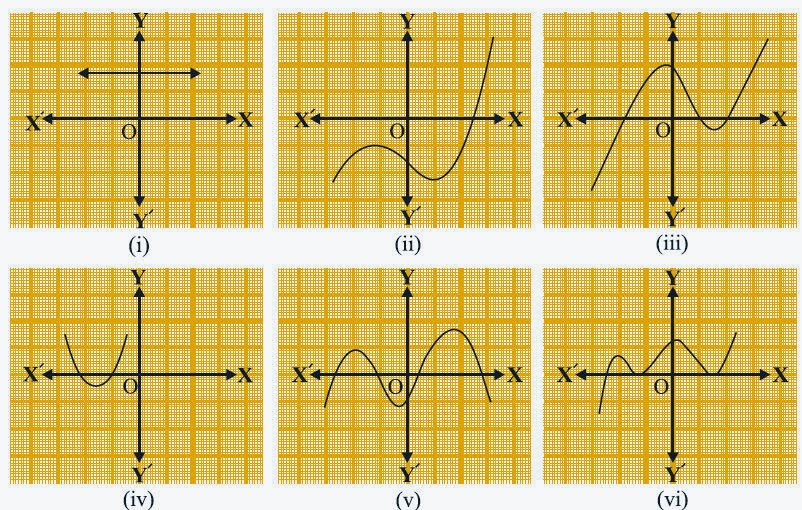## Saturday 25 April 2015

### CBSE Class 10 - Maths - Polynomials - NCERT Ex 2.1

POLYNOMIALS
NCERT Exercise 2.1 and other Q & A

Q1: The graphs of y = p(x) are given in below, for some polynomials p(x). Find the number of zeroes of p(x), in each case.

(i) As shown in the graph, it does not intersect x-axis. Hence it has no real zeroes.
(ii) From the graph, it intersects x-axis at one point. ∴ it has one real zero.
(iii) As the graph shows, it intersects x-axis at three points. ∴ the graph polynomial has three real zeroes.
(iv) From the graph, it is clear it intersects x-axis at two points. Hence it has two zeroes.
(v) As the graph shows, it meets x-axis at four points. ∴ it has four real zeroes.
(vi) As shown in the graph, it intersects x-axis at three points. ∴ it has three zeroes.

Q2(CBSE 2011): In the figure below the number of zeroes of y = f(x) are:

(a) 1
(b) 2
(c) 3
(d) 4

Answer: (c) 3. Since the graph intersects x-axis at three points.

Q3(CBSE 2011): The number of zeroes for the polynomial  y = p(x) from the given graph is :

(a) 3
(b) 1
(c) 2
(d) 0

Q4: Draw the graph of the quadratic polynomial p(x) = x2 +6x + 9 and find zeroes of it.

Answer: Given p(x) = x2 + 6x + 9
To find the zeroes of p(x), consider p(x) = 0   [Factor theorem]
∴ x2 + 6x + 9 = 0
⇒ (x + 3)2 = 0
⇒ x = -3.
∴ -3 is the zero of the polynomial.
For the graph, we take the following different values of x and y = p(x) = x2 + 6x + 9

 x -3 -2 -1 -4 y=p(x) 0 1 4 1

The graph plotted is shown below:

Comparing the quadratic polynomial as ax2 + bx + c, ∵ a =1 > 0, the graph parabola opens upwards(∪).#### 1 comment:

1.thanks for sharing the wonderful article

LATEST ADMIT CARDS EXAM HALL TICKETS